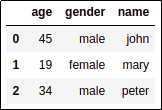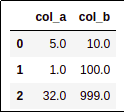Pandas Examples: Creating and Initializing DataFrames

Last updated:

Pandas version used: 0.24.2

There are many ways to build and initialize a pandas DataFrame. Here are some of the most common ones:

All examples can be found on this notebook

Create Dataframe from lists

Where each list represents one column.

import pandas as pd

names = ['john','mary','peter','gary','anne']
ages = [33,22,45,23,12]

df = pd.DataFrame({
'names':names,
'ages':ages
})
dfProbably the most straightforward
way to build dataframes

Create Dataframe from list of dicts

Use pd.DataFrame.from_records().

import pandas as pd

data_dicts = [
{'name':"john","gender":'male','age':45},
{'name':"mary", 'gender':"female",'age':19},
{'name':"peter",'gender':'male', 'age':34}
]

df = pd.DataFrame.from_records(data_dicts)
dfSince we didn't specify dtypes, they are automatically inferred from the data.

Create empty Dataframe and add rows one by one

Use append() with ignore_index=True.

import pandas as pd

# if you wish, you can set column names and dtypes here
df = pd.DataFrame()

# must reassign since the append method does not work in place
df = df.append({'col_a':5,'col_b':10}, ignore_index=True)
df = df.append({'col_a':1,'col_b':100}, ignore_index=True)
df = df.append({'col_a':32,'col_b':999}, ignore_index=True)

dfSince ignore_index is set, indices will start at 0